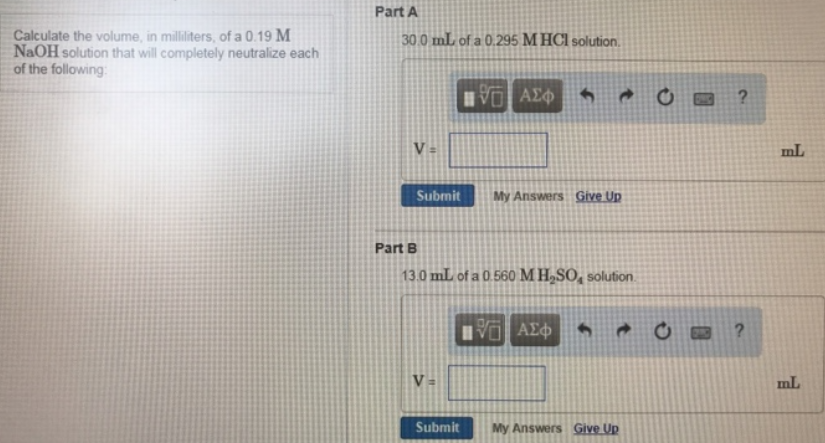# Problem: Calculate the volume, in milliliters, of a 0.19 M NaOH solution that will completely neutralize each of the following: Part A 30.0 mL of a 0.295 M HCl solution. Part B13.0 mL of a 0.560 M H2SO4 solution.

###### FREE Expert Solution
89% (161 ratings)###### Problem Details

Calculate the volume, in milliliters, of a 0.19 M NaOH solution that will completely neutralize each of the following:

Part A

30.0 mL of a 0.295 M HCl solution.

Part B

13.0 mL of a 0.560 M H2SO4 solution.